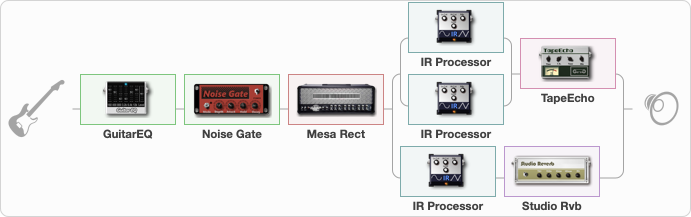# Mesa Boogie

Discussion in 'ToneLib-GFX presets' started by rivsegura, Dec 5, 2020.

1. Mesa Boogie

Preset name: My Distortion

Effects chain:Effect: "GuitarEQ" (Dynamics / Filter), active - "yes"
{
"160 Hz" = -4
"400 Hz" = -2
"800 Hz" = 2
"1.6 kHz" = 4
"3.2 kHz" = 0
"6.4 kHz" = 2
"12 kHz" = 6
"Level (dB)" = 0
}

Effect: "Noise Gate" (Dynamics / Filter), active - "yes"
{
"Mode" = Auto
"Depth" = 50
"Threshold" = 50
"Attack" = 0
"Hold" = 5
"Decay" = 250
}

Effect: "Mesa Rect" (Amp simulators), active - "yes"
{
"Gain" = 40
"Bass" = 50
"Middle" = 40
"Treble" = 50
"Presence" = 34
"Master" = 100
"Level (dB)" = 0
}

Effect: "Splitter" (Dynamics / Filter), active - "yes"
{
"A-Bypass" = Off
"A-Pan" = 0
"A-Level" = 55
"B-Bypass" = Off
"B-Pan" = 0
"B-Level" = 55

'A' branch:
{

Effect: "Splitter" (Dynamics / Filter), active - "yes"
{
"A-Bypass" = Off
"A-Pan" = 0
"A-Level" = 55
"B-Bypass" = Off
"B-Pan" = 0
"B-Level" = 55

'A' branch:
{

Effect: "IR Processor" (Cabinets), active - "yes"
{
"IR" = MesaRectifierV30s-SM57-Cap-0in
"Low Cut (Hz)" = 79
"Hi Cut (kHz)" = 10.0
"Mix" = 100
"Level (dB)" = 2
}
}
'B' branch:
{

Effect: "IR Processor" (Cabinets), active - "yes"
{
"IR" = MesaRectifierV30s-SM57-ConeEdge-3in
"Low Cut (Hz)" = 160
"Hi Cut (kHz)" = 20.0
"Mix" = 100
"Level (dB)" = -4
}
}
}

Effect: "TapeEcho" (Delay), active - "yes"
{
"Time" = 316
"Feedback" = 38
"Tone" = 65
"LoDamp" = 19
"Mix" = 65
}
}
'B' branch:
{

Effect: "IR Processor" (Cabinets), active - "yes"
{
"IR" = MesaRectifierV30s-KM84-Stereo-Room
"Low Cut (Hz)" = 80
"Hi Cut (kHz)" = 20.0
"Mix" = 100
"Level (dB)" = -10
}

Effect: "Studio Rvb" (Reverberation), active - "yes"
{
"Time" = 7.4
"PreDelay" = 32
"LoDamp" = 0
"HiDamp" = 12
"Mix" = 58
}
}
}

Note: You will need to download and install the ToneLib-GFX software to use the preset.

File size:
47.5 KB
Views:
2,491